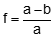### 1. Surfaces

There are two surfaces we routinely use with when performing a survey: physical earth and geoid.

#### a. Physical earth

This is the surface on which we set up equipment and perform measurements, Figure B-1.Figure B-1 Physical Earth

This is an irregular and non-mathematical surface so doesn't work well as a projection model.

#### b. Geoid

The geoid is an equipotential ("equal energy") surface defined by two primary forces: gravity and centrifugal, Figure B-2.Figure B-2 Geoid
##### 1. Gravity

Gravity, which pulls inward, is a function of mass. The earth is not a homogeneous solid, it has mass anomalies and a shifting molten interior. Instead of straight radial lines pointing to a single mass center, lines of gravity curve and deviate based on mass distribution. They are neither radial nor converge to a single point.

##### 2. Centrifugal force

Centrifugal force is caused by the earth's rotation and pushes outward. The further the surface is from the rotational axis, the greater the centrifugal force. It is greatest at the equation diminishing to zero at the poles. This tends to make the earth bulge at the equator and flatten at the poles.

When we set up equipment by centering a bubble, we are referencing ourselves to the geoid, Figure B-3.Figure B-3 Combined Earth and Geoid

Because of earth's internal dynamics the geoid is not uniform and changes over time. Although smoother than the physical earth, it is not a mathematical surface.

There's quite a bit more to earth dynamics than discussed here, but these are the primary characteristics about which we need to know.

### 2. Ellipsoid

An ellipse, Figure B-4, is a flattened circle having semi-major, a, and semi-minor, b, axes.Figure B-4 Ellipse

Geometric characteristics of an the ellipse are its flattening, f, first eccentricity, e, and second eccentricity, e'.Equation B-1Equation B-2Equation B-3

The ellipse is oriented so its semi-minor axis coincides with the Earth's polar axis, PP'. Spinning the ellipse around its semi-minor axis creates a 3D ellipsoid, Figure B-5.Figure B-5 Ellipsoid

An ellipsoid is used to build an earth model because it has the same general shape - bulging around the middle and flattened at top and bottom.

### 3. Almost There...

Now we have all the pieces. We just need to put them together to create a datum which is the next chapter.

Hits: 1895